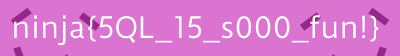/ BLUEHENSCTF  FORENSICS

# The Quantum Realm

Forensics! Stego! Look, they even gave us an image! You know the drill.``````\$ binwalk Antman.jpeg

--------------------------------------------------------------------------------
0             0x0             JPEG image data, EXIF standard
12            0xC             TIFF image data, big-endian, offset of first image directory: 8
9519          0x252F          Zip archive data, at least v2.0 to extract, compressed size: 10115, uncompressed size: 506796, name: could_this_be_it.txt
19786         0x4D4A          End of Zip archive, footer length: 22
``````

Too easy. Extraction time.

``````\$ binwalk Antman.jpeg -E
\$ cat _Antman.jpeg.extracted/could_this_be_it.txt | tr -cd '[:print:]\n' | base64 -d | head -15
45754(218, 116, 208)
(218, 116, 208)
(218, 116, 208)
(218, 116, 208)
(218, 116, 208)
(218, 116, 208)
(218, 116, 208)
(218, 116, 208)
(218, 116, 208)
(218, 116, 208)
(218, 116, 208)
(218, 116, 208)
(218, 116, 208)
(218, 116, 208)
(218, 116, 208)
``````

Not sure what’s going on with that initial number, but hey! It looks like we just have a list of RGB values. Except… what’s the dimensions of the image? That’s the eternal question. First, let’s see how many pixels we’re working with.

``````\$ cat _Antman.jpeg.extracted/could_this_be_it.txt | tr -cd '[:print:]\n' | base64 -d | wc -l
22400
``````

22400 pixels. Let’s see how many images there are with integral width and height that result in an image of that size.

``````\$ python3 -c 'print(len([(x, 22400) for x in range(1,11200) if 22400 % x == 0]))'
46
``````

I don’t know about you, but I can scroll through 46 images in a few seconds, so we might as well just try it out.

``````import sys
from PIL import Image

with open(sys.argv, 'r') as f:
# line format is (R, G, B) where R, G, B are in [0, 255]
lines = [line.strip().replace('(', '').replace(')', '').replace(',', ' ').split() for line in lines]
# convert to int
lines = [[int(x) for x in line] for line in lines]
# flatten list
lines = [item for sublist in lines for item in sublist]

i = 0
for x in range(1, 11200):
if 22400 % x == 0:
i += 1
im = Image.frombytes("RGB", (x, 22400 // x), bytes(lines))
im.save(f"{i:02}.png")
``````

Here’s a nice slider showing some of the frames.

Value:And that’s the Quantum Realm!#### squ1rrel team

Vanderbilt University's CTF team.